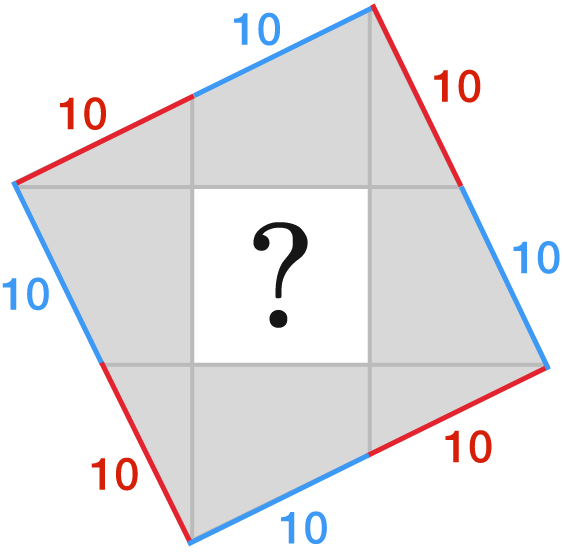A Square Inside A Square!

Geometry Level 2Line segments are drawn from the vertices of the large square to the midpoints of the opposite sides to form a smaller, white square.

If each red or blue line-segment measures $10$ m long, what is the area of the smaller, white square in m$^{2}?$

×